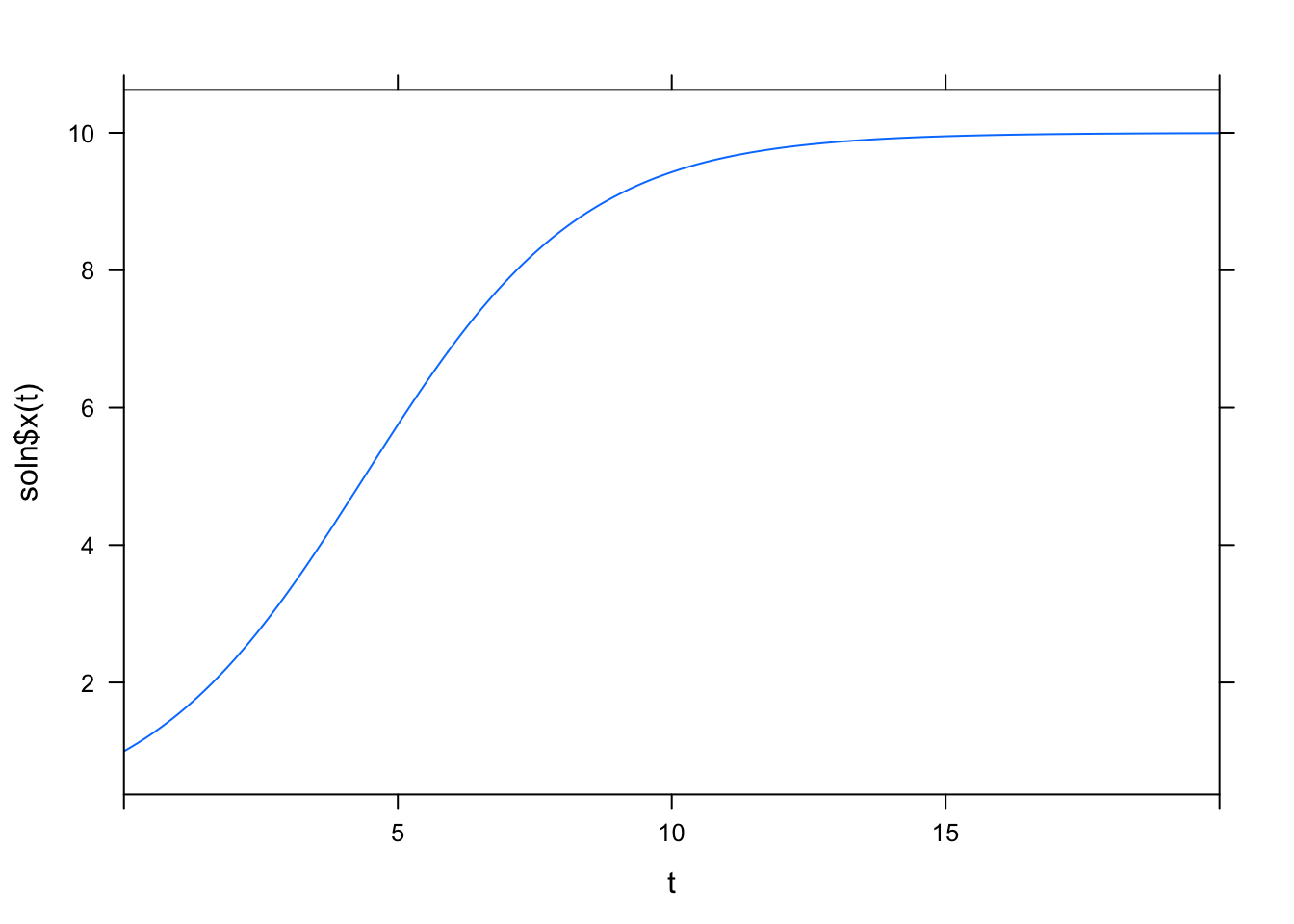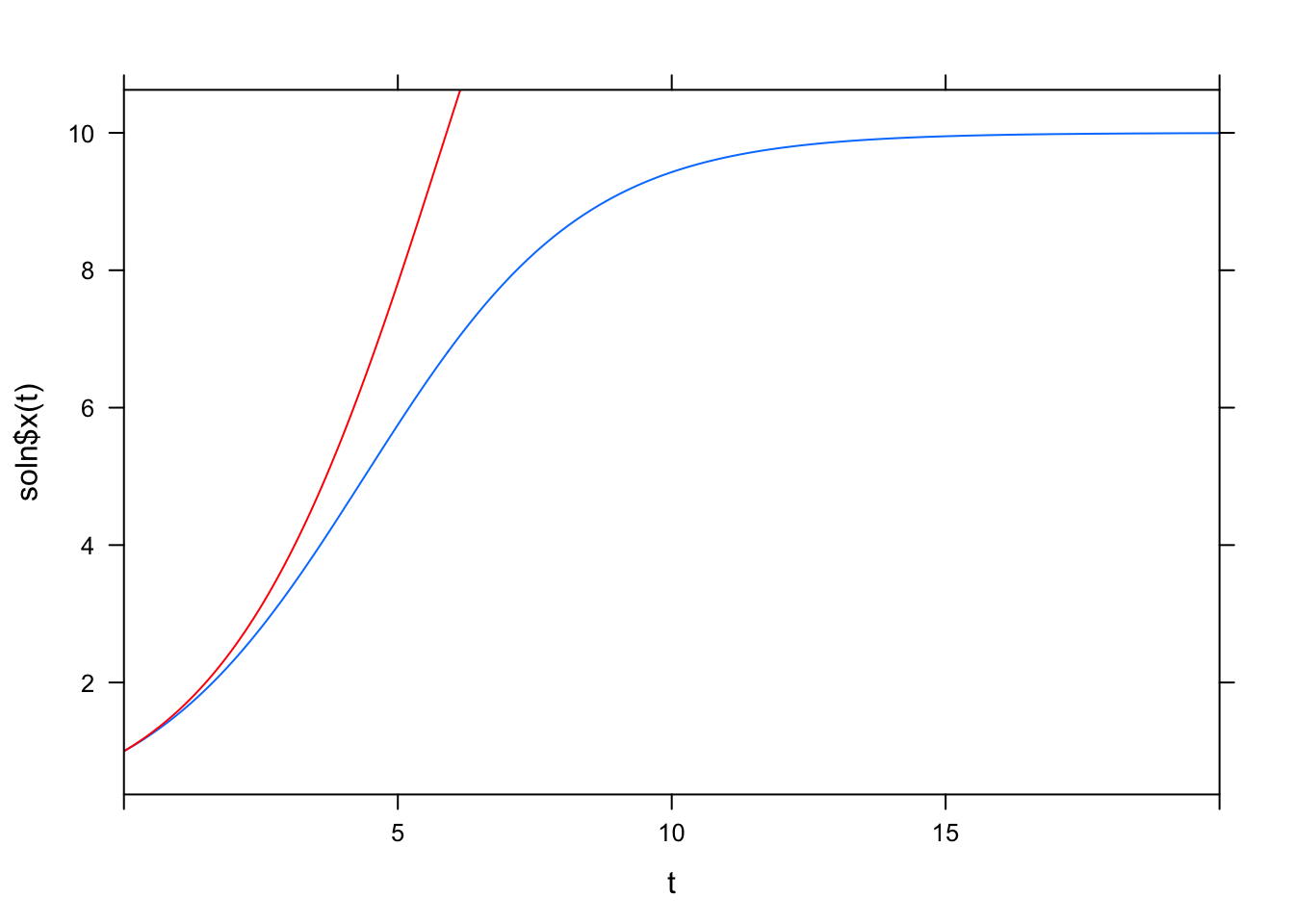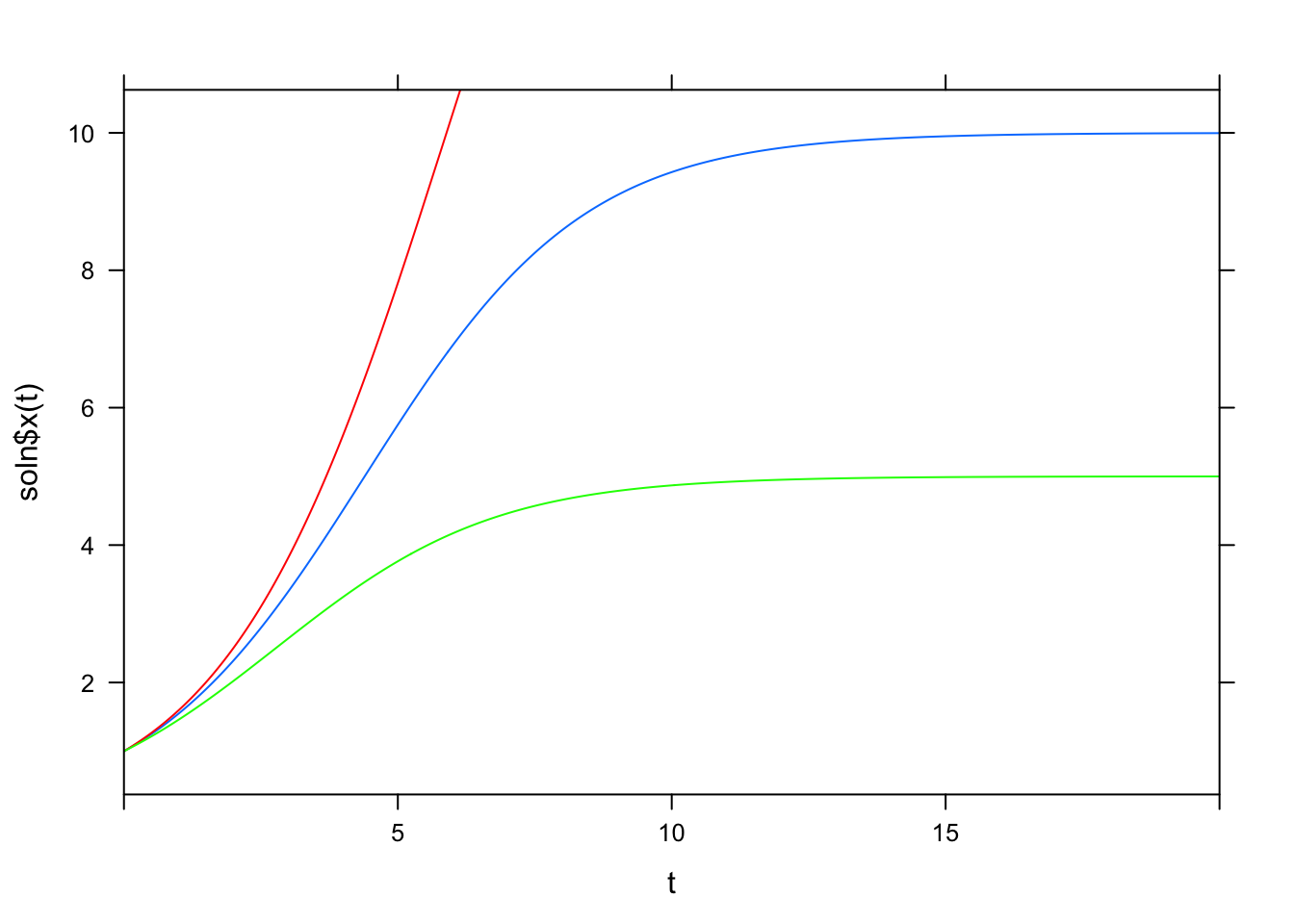We can use the mosaic package to do integration.

First run install.packages("mosaic") on your systems. You may need to do it 2x as it errored on a few systems last time.

You can test if it worked by doing:

library(mosaic)

and seeing if you get any errors

Now, say we have a simple logistic growth differential equation:

$$\frac{dx}{dt} = {r}{x}(1 - {x}/{K})$$

we can use the function integrateODE in the mosaic package to get the solution which we can then use for plotting/computation/etc. We need to give it the initial state for all the parameters and the duration (tdur) or range of time for the resultant function:

soln = integrateODE( dx ~ r * x * (1 - x/K), x=1, K=10, r=0.5,
tdur = list(from=0, to=20))

the pretty math formulas are easy to map into R syntax.

Now we can evaluate the function for individual or ranges of time:

print(soln$x(0)) ##  1 print(soln$x(1))
##  1.548281
print(soln$x(3)) ##  3.324279 print(soln$x(0:20))
##   1.000000 1.548281 2.319693 3.324279 4.508531 5.751209 6.905679
##   7.863017 8.584864 9.091066 9.428256 9.645239 9.781781 9.866497
##  9.918599 9.950469 9.969899 9.981721 9.988905 9.993268 9.995916

and, we can plot it, too.

plotFun(soln$x(t)~t, tlim=range(0, 20))and we can compare solutions as well. Here, we modify K a couple times: soln2 = integrateODE( dx ~ r * x * (1 - x/K), x=1, K=20, r=0.5, tdur = list(from=0, to=20)) soln3 = integrateODE( dx ~ r * x * (1 - x/K), x=1, K=5, r=0.5, tdur = list(from=0, to=20)) plotFun(soln$x(t)~t, tlim=range(0, 20))plotFun(soln2$x(t)~t, tlim=range(0, 20), add=TRUE, col="red")plotFun(soln3$x(t)~t, tlim=range(0, 20), add=TRUE, col="green")